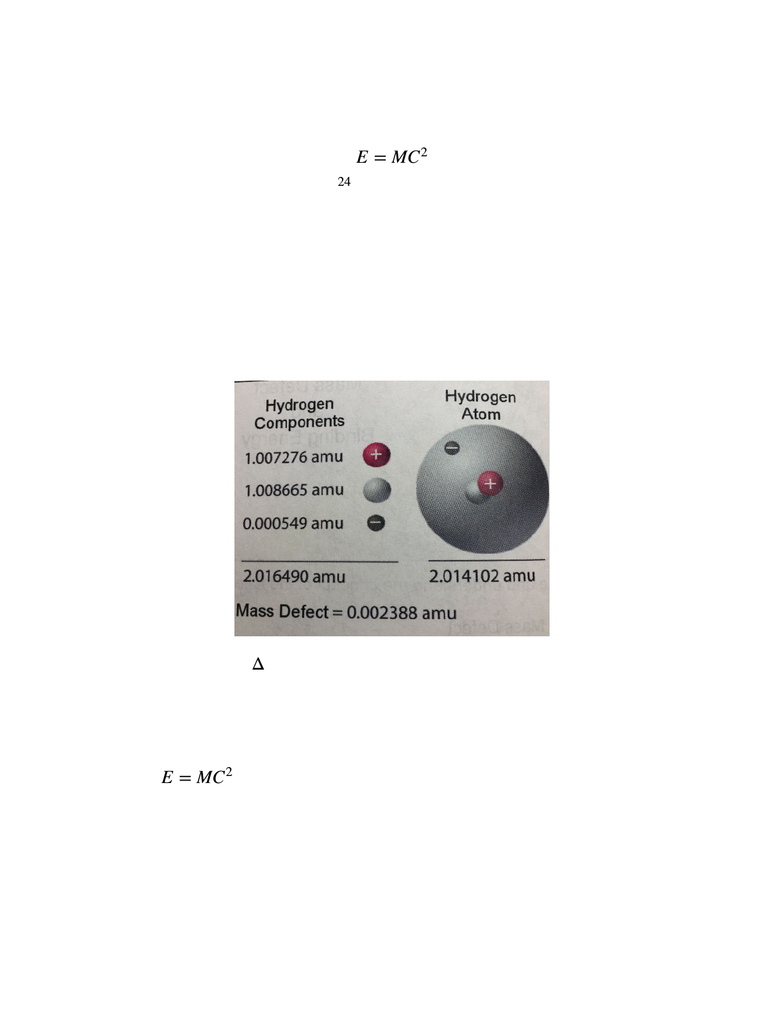Textbook Notes (280,000)
US (110,000)
TRCC (20)
NUC (10)
Abel (10)
Chapter 6

# NUC K117 Chapter 6: Mass Defect:Binding Energy

Department
Nuclear Engineering Technology
Course Code
NUC K117
Professor
Abel
Chapter
6

This preview shows half of the first page. to view the full 3 pages of the document.I. Mass Defect and Binding Energy!
1. All atoms are constructed on protons, neutrons, and electrons. Each “part” has
a mass and energy equivalent  !
 !
2. But, when an assembled atom is weighted, it weighs less than LESS than the
sum of its parts. The missing mass is referred to Mass Defect!
3. Mass Defect = Binding Energy!
The mass defect of .002388 amu equates to an energy level of 2.224 Mev!
So, energy of 2.224 Mev, is the energy present in the Strong Nuclear Force
that binds the atom together!
says that some of the assembled atom’s mass was converted to
energy!
4. Nuclear Fission!
E=MC2
1AMU = 1.6605 * 2024gm = 931.5 Mev
Electron = 9.11 * 1028gm = .511 Mev (.000549 A MU )
Proton = 1.673 * 1024gm = 938.2 Mev (1.007276 AMU )
Neutron = 1.675 * 1024gm = 939.6 Mev (1.008665 A MU )
Δ
E=MC2